﻿ 最优化算法中极小极大问题的教学思考 Thoughts on Teaching Min-Max Problem in Optimization Method

Creative Education Studies
Vol. 07  No. 05 ( 2019 ), Article ID: 32555 , 7 pages
10.12677/CES.2019.75107

Thoughts on Teaching Min-Max Problem in Optimization Method

Zhong Jin

College of Arts and Sciences, Shanghai Maritime University, Shanghai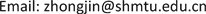Received: Sep. 26th, 2019; accepted: Oct. 9th, 2019; published: Oct. 16th, 2019ABSTRACT

Min-max problem model is a classical optimization model, as well as a way to solve multi objective programming, so it plays an important role in optimization method. By a simple example, min-max problem can be combined with multi objective programming and single objective programming, which would be useful for students to get an in-depth understanding of the content.

Keywords:Optimization Method, Min-Max Problem, Multi Objective Programming1. 引言

$\underset{x}{\mathrm{min}}\underset{i}{\mathrm{max}}{f}_{i}\left(x\right)$ .

${p}_{i}\left(i=1,2,\cdots ,m\right)$ ，平面上任一点x到各点的距离则分别为 $‖x-{p}_{i}‖\left(i=1,2,\cdots ,m\right)$ 。若以 $f\left(x\right)$ 表示x到m个点 ${p}_{i}$ 的最大距离，即 $f\left(x\right)=\underset{1\le i\le m}{\mathrm{max}}‖x-{p}_{i}‖$ ，则以x为圆心，以 $f\left(x\right)$ 为半径的圆盘能覆盖这m个点。于

$\underset{x\in {R}^{2}}{\mathrm{min}}f\left(x\right)=\underset{x\in {R}^{2}}{\mathrm{min}}\underset{1\le i\le m}{\mathrm{max}}‖x-{p}_{i}‖$ ,

$\underset{x\in D}{\mathrm{min}}\phi \left[f\left(x\right)\right]=\underset{x\in D}{\mathrm{min}}\underset{1\le i\le p}{\mathrm{max}}{f}_{i}\left(x\right)$ .

2. 模型建立

2.1. 多目标规划模型

$f\left(x\right)=\left({f}_{1}\left(x\right),{f}_{2}\left(x\right),{f}_{3}\left(x\right)\right)$ ，其中第一个目标是挖坑

${f}_{1}\left(x\right)=20{x}_{1}+10{x}_{4}$ ,

${f}_{2}\left(x\right)=30{x}_{2}+20{x}_{5}$ ,

${f}_{3}\left(x\right)=25{x}_{3}+15{x}_{6}$ ,

${x}_{1}+{x}_{2}+{x}_{3}\le 30$ ,

${x}_{4}+{x}_{5}+{x}_{6}\le 20$ ,

$\begin{array}{l}\mathrm{max}20{x}_{1}+10{x}_{4}\\ \mathrm{max}30{x}_{2}+20{x}_{5}\\ \mathrm{max}25{x}_{3}+15{x}_{6}\end{array}$

$\begin{array}{l}\text{s}\text{.t}\text{.}\text{ }25{x}_{3}+15{x}_{6}\le 20{x}_{1}+10{x}_{4},\\ \text{ }\text{ }25{x}_{3}+15{x}_{6}\le 30{x}_{2}+20{x}_{5},\\ \text{ }\text{ }{x}_{1}+{x}_{2}+{x}_{3}\le 30,\\ \text{ }\text{ }{x}_{4}+{x}_{5}+{x}_{6}\le 20,\\ \text{ }\text{ }其中{x}_{1},{x}_{2},{x}_{3},{x}_{4},{x}_{5},{x}_{6}都为整数\end{array}$

2.2. 极小极大问题模型

$\begin{array}{l}\text{ }\text{ }\mathrm{min}\underset{1\le i\le 3}{\mathrm{max}}{f}_{i}\left(x\right)\\ \text{s}\text{.t}\text{.}\text{ }{x}_{1}+{x}_{2}+{x}_{3}\le 30,\\ \text{ }\text{ }{x}_{4}+{x}_{5}+{x}_{6}\le 20,\\ \text{ }\text{ }其中{x}_{1},{x}_{2},{x}_{3},{x}_{4},{x}_{5},{x}_{6}都为整数\end{array}$

3. 模型的求解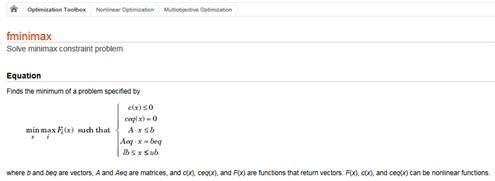Figure 1. The type of min-max problem which can be solved by fminimax

$\begin{array}{l}\text{ }\text{ }\mathrm{max}\text{\hspace{0.17em}}s\\ \text{s}\text{.t}\text{.}\text{ }\text{ }20{x}_{1}+10{x}_{4}\ge s,\\ \text{ }\text{ }30{x}_{2}+20{x}_{5}\ge s,\\ \text{ }\text{ }25{x}_{3}+15{x}_{6}\ge s,\end{array}$

$\begin{array}{l}{x}_{1}+{x}_{2}+{x}_{3}\le 30,\\ {x}_{4}+{x}_{5}+{x}_{6}\le 20,\\ 其中{x}_{1},{x}_{2},{x}_{3},{x}_{4},{x}_{5},{x}_{6}都为整数\end{array}$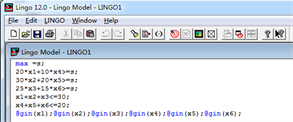Figure 2. The code of the model 3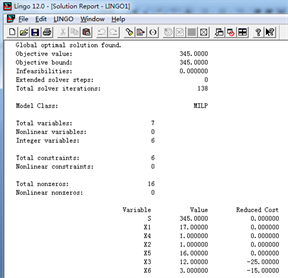Figure 3. The result of the model 3

4. 结果的验证和分析

4.1. 结果的验证

$\underset{x}{\mathrm{min}}\underset{i}{\mathrm{max}}{f}_{i}\left(x\right)=-\underset{x}{\mathrm{max}}\underset{i}{\mathrm{min}}\left(-{f}_{i}\left(x\right)\right)$ ,

$\begin{array}{l}\text{ }\text{ }-\mathrm{max}\underset{1\le i\le 3}{\mathrm{min}}\left(-{f}_{i}\left(x\right)\right)\\ \text{s}\text{.t}\text{.}\text{ }{x}_{1}+{x}_{2}+{x}_{3}\le 30\\ \text{ }\text{ }{x}_{4}+{x}_{5}+{x}_{6}\le 20\\ \text{ }\text{ }{x}_{1},{x}_{2},{x}_{3},{x}_{4},{x}_{5},{x}_{6}都为整数\end{array}$

$\begin{array}{l}\text{ }\text{ }-\mathrm{min}\text{\hspace{0.17em}}-s\\ \text{s}\text{.t}\text{.}\text{ }\text{ }-20{x}_{1}-10{x}_{4}\le -s,\\ \text{ }\text{ }-30{x}_{2}-20{x}_{5}\le -s,\\ \text{ }\text{ }-25{x}_{3}-15{x}_{6}\le -s,\end{array}$

$\begin{array}{l}{x}_{1}+{x}_{2}+{x}_{3}\le 30,\\ {x}_{4}+{x}_{5}+{x}_{6}\le 20,\\ 其中{x}_{1},{x}_{2},{x}_{3},{x}_{4},{x}_{5},{x}_{6}都为整数\end{array}$Figure 4. The code of model 5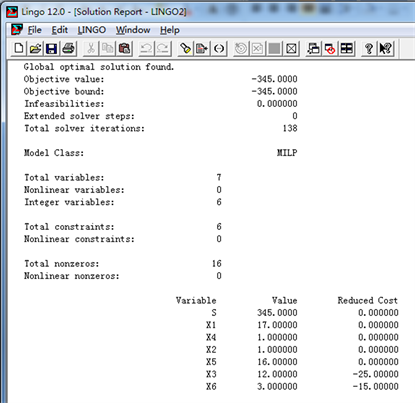Figure 5. The result of model 5

4.2. 结果的分析

$\mathrm{max}s=-\mathrm{min}\text{\hspace{0.17em}}-s$ ,

$20{x}_{1}+10{x}_{4}\ge s$ , $30{x}_{2}+20{x}_{5}\ge s$ , $25{x}_{3}+15{x}_{6}\ge s$ ,

$-20{x}_{1}-10{x}_{4}\le -s$ , $-30{x}_{2}-20{x}_{5}\le -s$ , $-25{x}_{3}-15{x}_{6}\le -s$ ,

5. 总结

Thoughts on Teaching Min-Max Problem in Optimization Method[J]. 创新教育研究, 2019, 07(05): 628-634. https://doi.org/10.12677/CES.2019.75107

1. 1. 施光燕, 钱伟懿, 庞丽萍. 最优化算法[M]. 第二版. 北京: 高等教育出版社, 2007.

2. 2. 孙文瑜, 徐成贤, 朱德通. 最优化方法[M]. 第二版. 北京: 高等教育出版社, 2010.

3. 3. 李昌兴, 徐迈, 惠莉萍. 非线性极小极大问题的分数阶粒子群算法[J]. 西安邮电大学学报, 2018, 23(6): 81-86+93.

4. 4. 赵奇, 张燕. 极小极大问题的非单调滤子算法[J]. 运筹学学报, 2012, 16(2): 91-104.

5. 5. 贺莉, 刘庆怀. 多目标优化理论与连续化方法[M]. 北京: 科学出版社, 2015.